# Gauss-Kronrod quadrature formula

A quadrature formula of highest algebraic accuracy of the type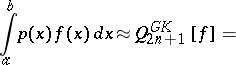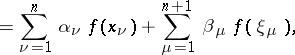whereare fixed, being the nodes of the Gauss quadrature formula, andis a weight function (see Quadrature formula). Depending on, its algebraic accuracy is at least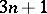, but may be higher (see Quadrature formula of highest algebraic accuracy). For the special case, which is most important for practical calculations, the algebraic accuracy is preciselyifis even and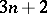ifis odd [a7].

The pairprovides an efficient means for the approximate calculation of definite integrals with practical error estimate, and hence for adaptive numerical integration routines (cf. also Adaptive quadrature). Gauss–Kronrod formulas are implemented in the numerical software package QUADPACK [a6], and they are presently (1998) the standard method in most numerical libraries.

The nodesof the Gauss–Kronrod formula are the zeros of the Stieltjes polynomial, which satisfies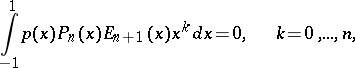whereis the system of orthogonal polynomials with respect to(cf. also Stieltjes polynomials). An iteration of these ideas leads to a nested sequence of Kronrod–Patterson formulas (cf. Kronrod–Patterson quadrature formula).

For several special cases of weight functions, the Stieltjes polynomials have real roots insidewhich interlace with the zeros of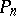. In particular, this is known for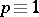, and in this case also the weights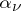andof the Gauss–Kronrod formulas are positive. These facts are not necessarily true in general, see [a3], [a4], [a5] for surveys. The nodes and weights of Gauss–Kronrod formulas forare distributed very regularly (see also Stieltjes polynomials for asymptotic formulas and inequalities).

Error bounds for Gauss–Kronrod formulas have been given in [a2]. It is known that for smooth (i.e. sufficiently often differentiable) functions, Gauss–Kronrod formulas are significantly inferior to the Gauss quadrature formulas (cf. Gauss quadrature formula) which use the same number of nodes (see [a2]). Cf. also Stopping rule for practical error estimation with the Gauss and other quadrature formulas.